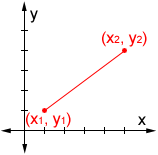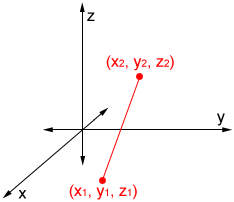﻿ Distance Calculator - A.Tools

# Distance Calculator

Point1=( ), ( )
Point2=( ), ( )The Distance between 2 Points ({{point2D1.x}},{{point2D1.y}}) ({{point2D2.x}},{{point2D2.y}}):
{{distance2D}}
Point1=( ), ( ), ( )
Point2=( ), ( ), ( )The Distance between 2 Points ({{point3D1.x}},{{point3D1.y}},{{point3D1.z}}) ({{point3D2.x}},{{point3D2.y}},{{point3D2.z}}):
{{distance3D}}#### Perimeter Calculator

Perimeter Calculator supports perimeter calculations for common geometric figures such as Circular, Sector, Ellipse, Triangle, Square, Rectangle, and Parallelogram. You can use different formulas and methods to calculate the perimeter of these geometric figures depending on the shape and the given information.#### Area Calculator

Area Calculator supports area calculations for common geometric figures such as Circular, Sector, Ring, Ellipse, Triangle, Square, Rectangle, Parallelogram and Trapezoid.#### Surface Area Calculator

Surface Area Calculator supports surface area calculations for common geometric figures such as Sphere, Spherical Sector, Segment, Cylinder, Hollow Cylinder(Pipe), Oblique Straight Cylinder, Cone, Circular Truncated Cone, Ellipsoid, Triangle Prism, Pyramid, Cube, Cuboid, Torus and Sphere Belt.#### Volume Calculator

Volume Calculator supports volume calculations for common geometric figures such as Sphere, Spherical Sector, Segment, Cylinder, Hollow Cylinder(Pipe), Oblique Straight Cylinder, Cone, Circular Truncated Cone, Ellipsoid, Triangle Prism, Pyramid, Cube, Cuboid, Torus and Sphere Belt.